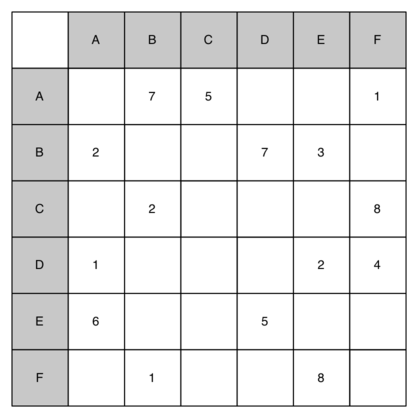# 9.24. Discussion Questions¶

1. Draw the graph corresponding to the following adjacency matrix.1. Draw the graph corresponding to the following list of edges.

from

to

cost

1

2

10

1

3

15

1

6

5

2

3

7

3

4

7

3

6

10

4

5

7

6

4

5

5

6

13

2. Ignoring the weights, perform a breadth first search on the graph from the previous question.

3. What is the Big-O running time of the buildGraph function?

4. Derive the Big-O running time for the topological sort algorithm.

5. Derive the Big-O running time for the strongly connected components algorithm.

6. Show each step in applying Dijkstra’s algorithm to the graph shown above.

7. Using Prim’s algorithm, find the minimum weight spanning tree for the graph shown above.

8. Draw a dependency graph illustrating the steps needed to send an email. Perform a topological sort on your graph.

9. Derive an expression for the base of the exponent used in expressing the running time of the knights tour.

10. Explain why the general DFS algorithm is not suitable for solving the knights tour problem.

11. What is the Big-O running time for Prim’s minimum spanning tree algorithm?